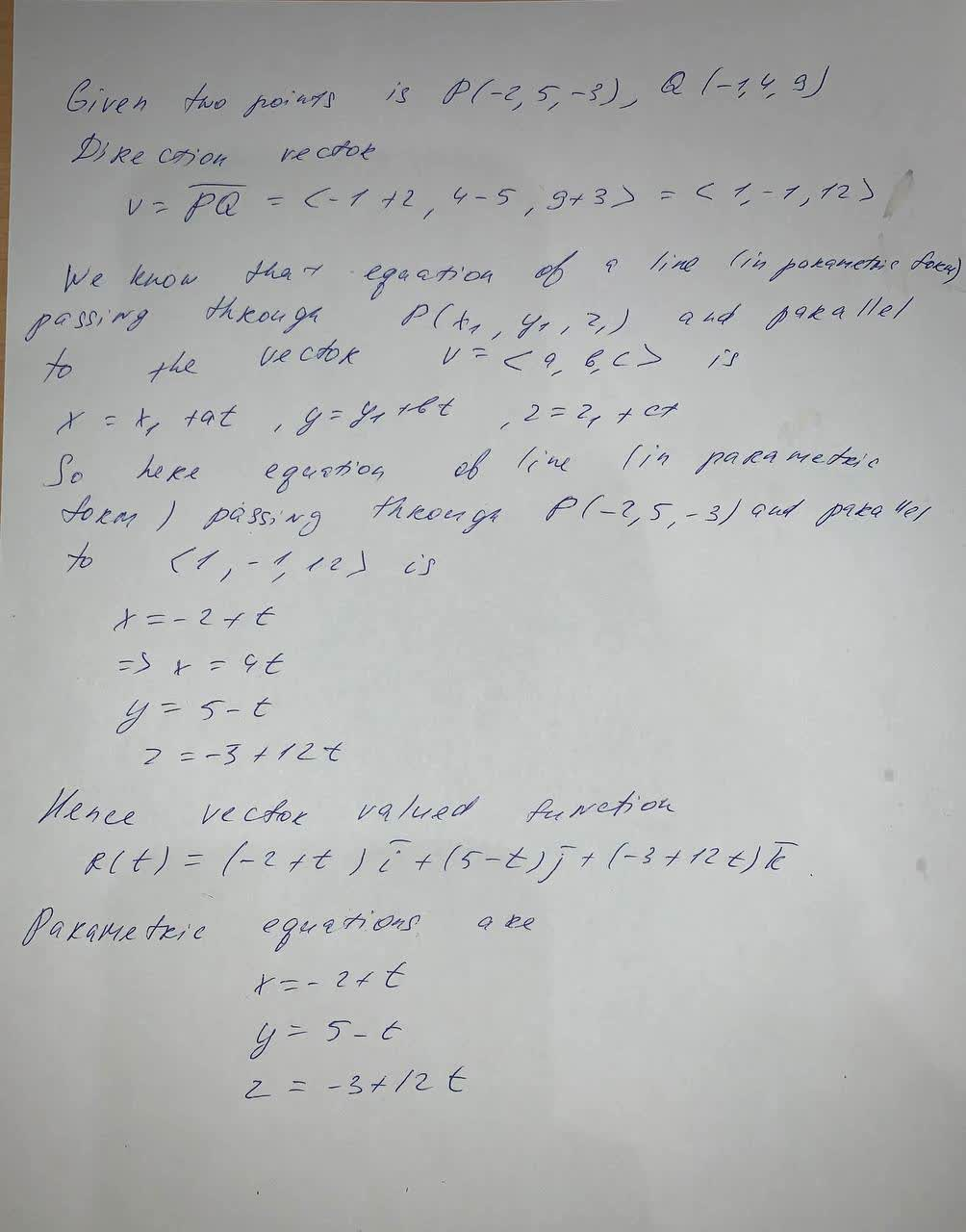Writing a Vector-Valued function in exercise , represent the line segment from P to Q by a vector-valued function and by a set of parametric equations: P(-2,5,-3),Q(-1,4,9)rocedwrp 2021-08-29 Answered
Writing a Vector-Valued function in exercise , represent the line segment from P to Q by a vector-valued function and by a set of parametric equations:
P(-2,5,-3),Q(-1,4,9)

• Questions are typically answered in as fast as 30 minutes

Solve your problem for the price of one coffee

• Math expert for every subject
• Pay only if we can solve itopsadnojD

To represent the line segment from P to Q by a vector-valued function you need